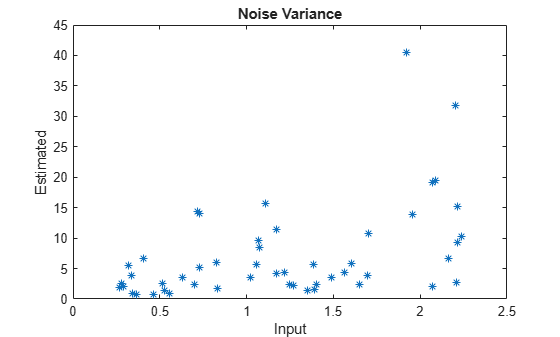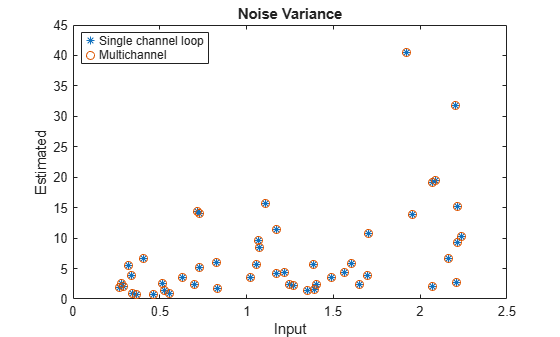# aryule

Autoregressive all-pole model parameters — Yule-Walker method

## Syntax

``a = aryule(x,p)``
``[a,e,rc] = aryule(x,p)``

## Description

example

````a = aryule(x,p)` returns the normalized autoregressive (AR) parameters corresponding to a model of order `p` for the input array `x`.```

example

````[a,e,rc] = aryule(x,p)` also returns the estimated variance, `e`, of the white noise input and the reflection coefficients, `rc`.```

## Examples

collapse all

Use a vector of polynomial coefficients to generate an AR(4) process by filtering 1024 samples of white noise. Reset the random number generator for reproducible results. Use the Yule-Walker method to estimate the coefficients.

```rng default A = [1 -2.7607 3.8106 -2.6535 0.9238]; y = filter(1,A,0.2*randn(1024,1)); arcoeffs = aryule(y,4)```
```arcoeffs = 1×5 1.0000 -2.7262 3.7296 -2.5753 0.8927 ```

Generate 50 realizations of the process, changing each time the variance of the input noise. Compare the Yule-Walker-estimated variances to the actual values.

```nrealiz = 50; noisestdz = rand(1,nrealiz)+0.5; randnoise = randn(1024,nrealiz); noisevar = zeros(1,nrealiz); for k = 1:nrealiz y = filter(1,A,noisestdz(k) * randnoise(:,k)); [arcoeffs,noisevar(k)] = aryule(y,4); end plot(noisestdz.^2,noisevar,'*') title('Noise Variance') xlabel('Input') ylabel('Estimated')```Repeat the procedure using the function's multichannel syntax.

```Y = filter(1,A,noisestdz.*randnoise); [coeffs,variances] = aryule(Y,4); hold on plot(noisestdz.^2,variances,'o') hold off legend('Single channel loop','Multichannel','Location','best')```Use a vector of polynomial coefficients to generate an AR(2) process by filtering 1024 samples of white noise. Reset the random number generator for reproducible results.

```rng default y = filter(1,[1 -0.75 0.5],0.2*randn(1024,1));```

Use the Yule-Walker method to fit an AR(10) model to the process. Output and plot the reflection coefficients. Only the first two coefficients lie outside the 95% confidence bounds, indicating that an AR(10) model significantly overestimates the time dependence in the data. See AR Order Selection with Partial Autocorrelation Sequence for more details.

```[ar,nvar,rc] = aryule(y,10); stem(rc) xlim([0 11]) conf95 = sqrt(2)*erfinv(0.95)/sqrt(1024); [X,Y] = ndgrid(xlim,conf95*[-1 1]); hold on plot(X,Y,'--r') hold off title('Reflection Coefficients')```## Input Arguments

collapse all

Input array, specified as a vector or matrix.

Example: `filter(1,[1 -0.75 0.5],0.2*randn(1024,1))` specifies a second-order autoregressive process.

Data Types: `single` | `double`
Complex Number Support: Yes

Model order, specified as a positive integer scalar. `p` must be less than the number of elements or rows of `x`.

Data Types: `single` | `double`

## Output Arguments

collapse all

Normalized autoregressive parameters, returned as a vector or matrix. If `x` is a matrix, then each row of `a` corresponds to a column of `x`. `a` has `p` + 1 columns and contains the AR system parameters, A(z), in descending powers of z.

White noise input variance, returned as a scalar or row vector. If `x` is a matrix, then each element of `e` corresponds to a column of `x`.

Reflection coefficients, returned as a column vector or matrix. If `x` is a matrix, then each column of `rc` corresponds to a column of `x`. `rc` has `p` rows.

collapse all

### AR(p) Model

In an AR model of order p, the current output is a linear combination of the past p outputs plus a white noise input.

The weights on the p past outputs minimize the mean squared prediction error of the autoregression. If y(n) is the current value of the output and x(n) is a zero-mean white noise input, the AR(p) model is

`$\sum _{k=0}^{p}a\left(k\right)y\left(n-k\right)=x\left(n\right).$`

### Reflection Coefficients

The reflection coefficients are the partial autocorrelation coefficients scaled by –1.

The reflection coefficients indicate the time dependence between y(n) and y(n – k) after subtracting the prediction based on the intervening k – 1 time steps.

## Algorithms

`aryule` uses the Levinson-Durbin recursion on the biased estimate of the sample autocorrelation sequence to compute the parameters.

 Hayes, Monson H. Statistical Digital Signal Processing and Modeling. New York: John Wiley & Sons, 1996.